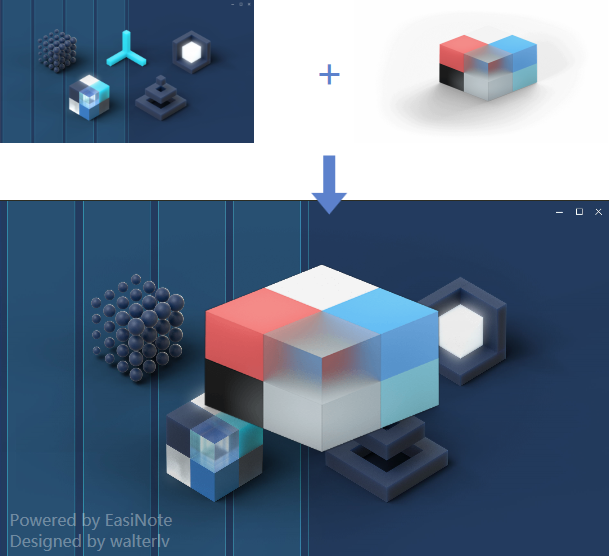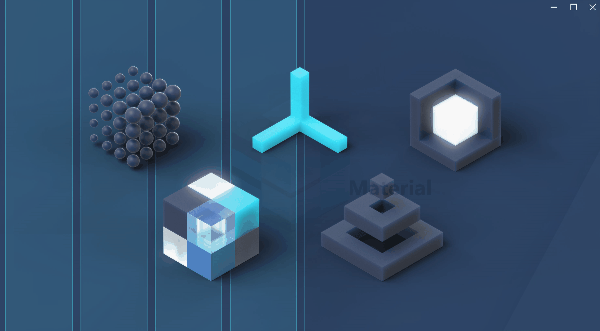# 透明度叠加算法：如何计算半透明像素叠加到另一个像素上的实际可见像素值（附 WPF 和 HLSL 的实现）

## 算法

float r = (foreground.r * alpha) + (background.r * (1.0 - alpha));


## 在 C# 代码中实现

for (int i = 0; i + 4 < length; i = i + 4)
{
var backB = background[i];
var backG = background[i + 1];
var backR = background[i + 2];
var foreB = foreground[i];
var foreG = foreground[i + 1];
var foreR = foreground[i + 2];
double alpha = foreground[i + 3];

blue = 0;

output[i] = (foreB * alpha) + (backB * (1.0 - alpha));
output[i + 1] = (foreG * alpha) + (backG * (1.0 - alpha));
output[i + 2] = (foreR * alpha) + (backR * (1.0 - alpha));
output[i + 3] = 1.0;
}


## 在像素着色器中实现

/// <description>透明度叠加效果。</description>

sampler2D Background : register(s0);
sampler2D Foreground : register(s1);

float4 main(float2 uv : TEXCOORD) : COlOR
{
float4 background = tex2D(Background, uv);
float4 foreground = tex2D(Foreground, uv);
float alpha = foreground.a;

float r = (foreground.r * alpha) + (background.r * (1.0 - alpha));
float g = (foreground.g * alpha) + (background.g * (1.0 - alpha));
float b = (foreground.b * alpha) + (background.b * (1.0 - alpha));
float a = 1.0;

return float4(r, g, b, a);
}/// <description>透明度叠加效果。</description>

/// <type>Double</type>
/// <summary>采样 2 的叠加透明度。</summary>
/// <minValue>0.0</minValue>
/// <maxValue>1.0</maxValue>
/// <defaultValue>0.75</defaultValue>
float Alpha : register(C0);

sampler2D Background : register(s0);
sampler2D Foreground : register(s1);

float4 main(float2 uv : TEXCOORD) : COlOR
{
float4 background = tex2D(Background, uv);
float4 foreground = tex2D(Foreground, uv);
float alpha = Alpha;

float r = (foreground.r * alpha) + (background.r * (1.0 - alpha));
float g = (foreground.g * alpha) + (background.g * (1.0 - alpha));
float b = (foreground.b * alpha) + (background.b * (1.0 - alpha));
float a = 1.0;

return float4(r, g, b, a);
}本作品采用 知识共享署名-非商业性使用-相同方式共享 4.0 国际许可协议 进行许可。欢迎转载、使用、重新发布，但务必保留文章署名 吕毅 （包含链接： https://blog.walterlv.com ），不得用于商业目的，基于本文修改后的作品务必以相同的许可发布。如有任何疑问，请 与我联系 (walter.lv@qq.com)